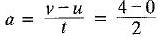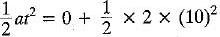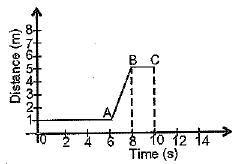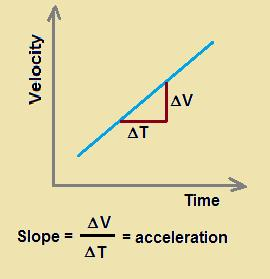Courses

# MCQ : Motion - 2

## 10 Questions MCQ Test Science Class 9 | MCQ : Motion - 2

Description
This mock test of MCQ : Motion - 2 for Class 9 helps you for every Class 9 entrance exam. This contains 10 Multiple Choice Questions for Class 9 MCQ : Motion - 2 (mcq) to study with solutions a complete question bank. The solved questions answers in this MCQ : Motion - 2 quiz give you a good mix of easy questions and tough questions. Class 9 students definitely take this MCQ : Motion - 2 exercise for a better result in the exam. You can find other MCQ : Motion - 2 extra questions, long questions & short questions for Class 9 on EduRev as well by searching above.
QUESTION: 1

### Equations of motion can be used for a body having______.

Solution:

The equations of motion are applicable only when the body moves with uniform acceleration. A body under constant acceleration is uniformly accelerated motion.

QUESTION: 2

### The magnitude of the displacement of an object is always______.

Solution:
• Displacement is the shortest straight line distance between the initial and final position. This means that there doesn't exist any other path which is shorter than it.
• If the object travels with that shortest straight-line path then the magnitude of distance and displacement would be the same.
• If the object doesn't do so and travels with any other paths then as the other path is not the shortest path, so it will be larger than that of displacement. So if the object travels with this path then the magnitude of displacement will be smaller than the distance.

Thus, the magnitude of displacement may be equal to or smaller than that of distance.

QUESTION: 3

### For a body starting from rest, the displacement in 10 seconds, when it acquires 4 ms-1 in 2 seconds is______.

Solution:

v = u + at
or= 2m/s2
s = ut += 100 m

QUESTION: 4

Retardation of a body is expressed in________.

Solution:
• Retardation, actually, is acceleration that acts opposite to the direction of motion of an object.
• So, under retardation, an object accelerates away from its direction of motion, so its velocity in that direction decreases.
• The SI unit of retardation, which is just an oppositely directed acceleration, is m/s2.
QUESTION: 5

The v-t graph for the x-t graph given here is best drawn asSolution:
QUESTION: 6

On a velocity-time graph, a horizontal straight line corresponds to motion at_______.

Solution:

It is horizontal so, angle is zero and also acceleration is zero. Hence constant velocity.

QUESTION: 7

For a uniformly accelerated body with initial and final velocities as u and v ms-1, the average velocity is _______.

Solution:

Average velocity of an object is equal to its final velocity plus initial velocity, divided by 2.
Therefore, the answer is, (u + v) / 2

QUESTION: 8

Which of the following graphs show that the body is at rest?

Solution:

In figure A, we can see that the object continues to remain at a fixed place while the time keeps running. So, the object is at rest, because the object does not change its position with time.

QUESTION: 9

The area below v - t graph is a measure of_______.

Solution:

"Displacement" is the quantity that is measured by the area occupied under the velocity-time graph. Moving something or someone from its place or position is called displacement.

QUESTION: 10

The slope of velocity-time graph gives_______.

Solution:The slope of a velocity-time graph represents the acceleration of the object. So, the value of the slope at a particular time represents the acceleration of the object at that instant.